# 距离和相似度度量方法

## 不同距离度量的应用场景

### 距离度量公理Axioms of Distance Measures

1) d(x,y) = 0  iff x = y                  // 到自己的距离为0
2) d(x,y) >= 0                  // 距离非负
3) d(x,y) = d(y,x)                   // 对称性: 如果 A 到 B 距离是 a，那么 B 到 A 的距离也应该是 a
4) d(x,k)+ d(k,y) >= d(x,y)    // 三角形法则triangle inequality: (两边之和大于第三边)

Note: iff =  if and only if

[熵与互信息 ]

## 文本相似度量方法一览

1. 字符串/序列
2. 包含较多文本内容的文档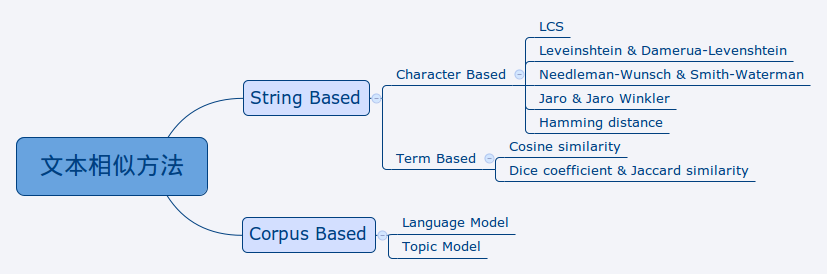Note: lz这里LCS也可以认为就是编辑距离吧。

1. String Based，即基于待比较的文本本身中的信息，该类方法评估的是”词法“上的相似性，或说朴素的相似性
2. Corpus Based，即基于一个较大的文本集合中的信息，该类方法评估的是“语义”上的相似性

# 欧氏距离度量

## 范数Norm

Lr范数就是x,y每个维度差距上取r次方加和后再开r次方根。Lr norm: what you get by taking the rth power of the differences, summing and taking the rth root.

L1范数：向量各个元素绝对值之和，Manhattan distance。L2范数：向量各个元素的平方求和然后求平方根，也叫欧式范数、欧氏距离。Lp范数：向量各个元素绝对值的p次方求和然后求1/p次方。L∞范数：向量各个元素求绝对值，最大那个元素的绝对值。也就是x，y在任意维度上差距最大的那个值。### 小示例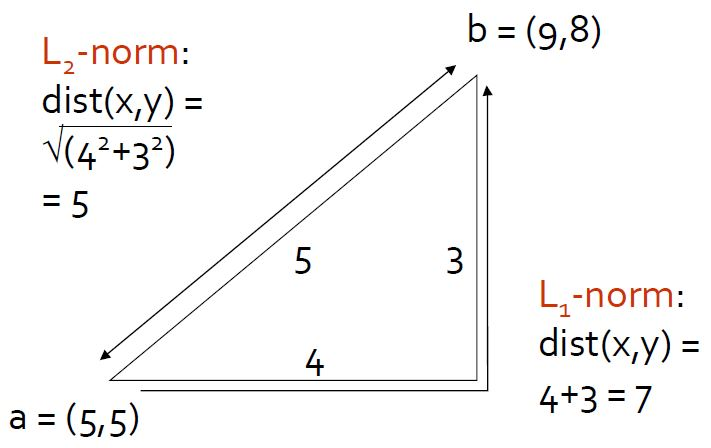## Euclidean distance Vs. Non-Euclidean distance 欧氏距离对比非欧氏距离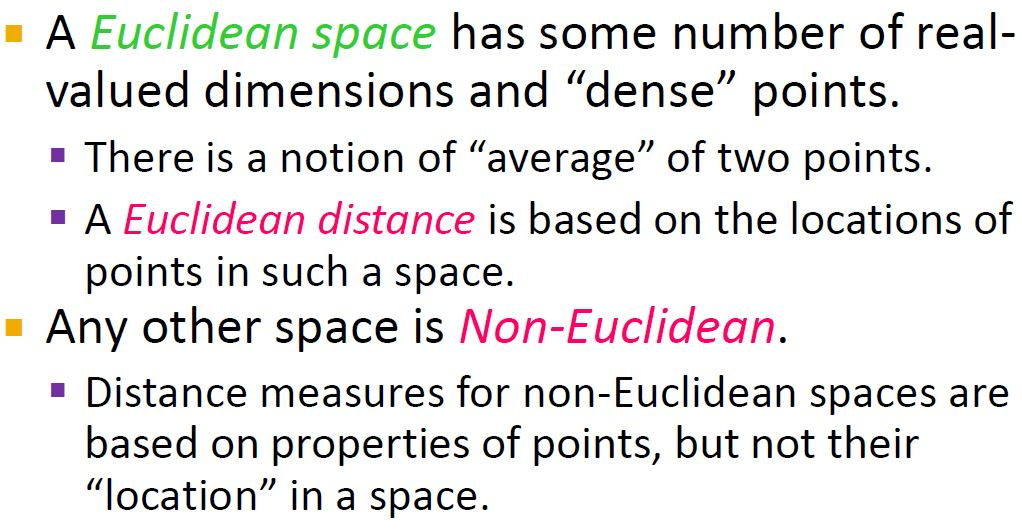dense: 给定两个点，它们的均值也是这个空间的一个点。And there is no reasonable notion of the average of points in the space.欧氏距离可以计算average，但是非欧氏距离却不一定。

# 非欧氏距离度量

## Jaccard相似度

Note: Jaccard distance = 1 - Jaccard similarity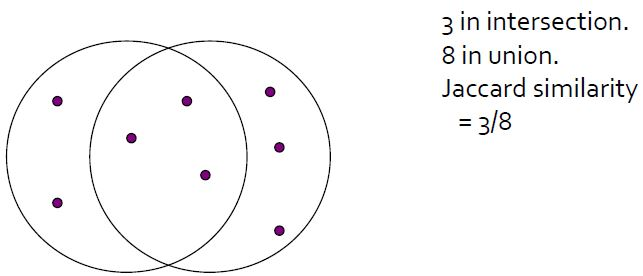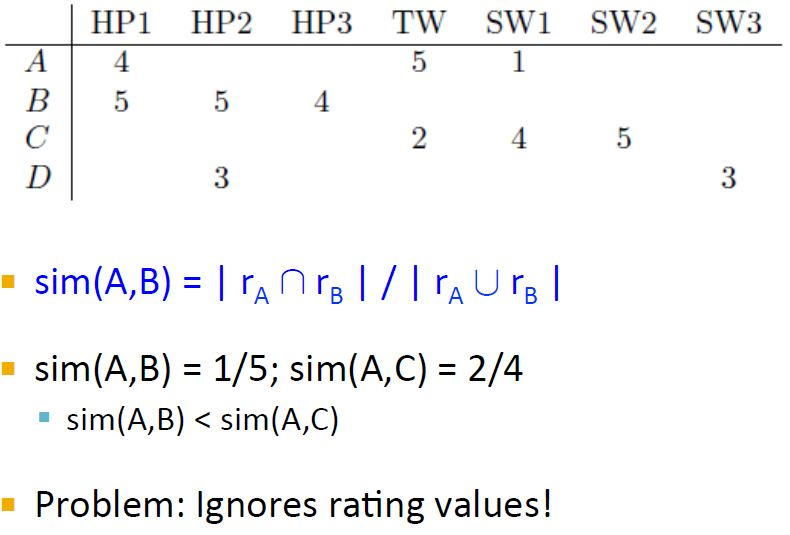Jaccard相似度没有考虑评分的大小。

### Dice's CoefficientDice 系数和 Jaccard 相似性起初被用于生态学上，作为一种判断物种间相似性的方法。在生态学上，要比较两个物种间相似程度时，通常会对该物种的特性进行采样，最后得到各自的特性集合，而 Dice 系数和 Jaccard 相似性都是通过比较两者之间的 共有特性 占比来度量相似性的，因此这两种方法都不是很关心每个 "Term" 的具体量，只是关心有没有某个 "Term"。

### 广义Jaccard相似度|Tanimoto系数

EJ(A,B)=(A*B)/(||A||^2+||B||^2-A*B)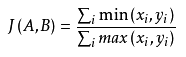## Cosine余弦相似度/向量内积

1. 两个向量间的余弦值可以很容易地通过使用欧几里得点积和量级公式推导$\mathbf{a}\cdot\mathbf{b}=\left\|\mathbf{a}\right\|\left\|\mathbf{b}\right\|\cos\theta$$\text{similarity} = \cos(\theta) = {A \cdot B \over \|A\| \|B\|} = \frac{ \sum\limits_{i=1}^{n}{A_i \times B_i} }{ \sqrt{\sum\limits_{i=1}^{n}{(A_i)^2}} \times \sqrt{\sum\limits_{i=1}^{n}{(B_i)^2}} }$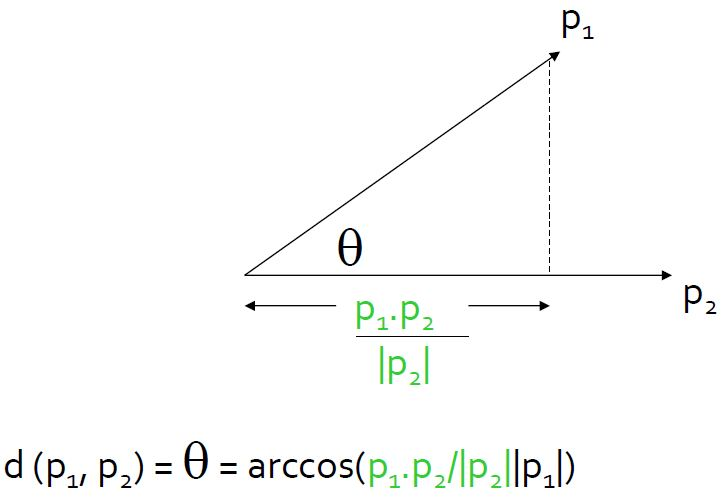Note:  if you project P1 onto P2,the length of the projection is the dot product, divided by the length of P2.Then the cosine of the angle between them is the ratio of adjacent(the dot product divided by P2) over hypotenuse(斜边, the length of P1).

[余弦相似性]

2. 向量内积是线性代数里最为常见的计算，实际上它还是一种有效并且直观的相似性测量手段。向量内积的定义如下：$\text{similarity} = \cos(\theta) = {A \cdot B \over \|A\| \|B\|} = \frac{ \sum\limits_{i=1}^{n}{A_i \times B_i} }{ \sqrt{\sum\limits_{i=1}^{n}{(A_i)^2}} \times \sqrt{\sum\limits_{i=1}^{n}{(B_i)^2}} }$$\text{similarity} = \cos(\theta) = {A \cdot B \over \|A\| \|B\|} = \frac{ \sum\limits_{i=1}^{n}{A_i \times B_i} }{ \sqrt{\sum\limits_{i=1}^{n}{(A_i)^2}} \times \sqrt{\sum\limits_{i=1}^{n}{(B_i)^2}} }$

cosine similarity的一个扩展是

## Tonimoto系数## 皮尔逊相关系数（Pearson correlation）

{也叫Centered Cosine，有时候也直接叫相关系数}

{数值型数据的相关性分析Correlation Analysis (Numerical Data)，从下面这个公式看出，它其实就是将数据归一化（数据减去其对应均值）后进行cosine相似度计算，所以叫centered cosine}$\text{similarity} = \cos(\theta) = {A \cdot B \over \|A\| \|B\|} = \frac{ \sum\limits_{i=1}^{n}{A_i \times B_i} }{ \sqrt{\sum\limits_{i=1}^{n}{(A_i)^2}} \times \sqrt{\sum\limits_{i=1}^{n}{(B_i)^2}} }$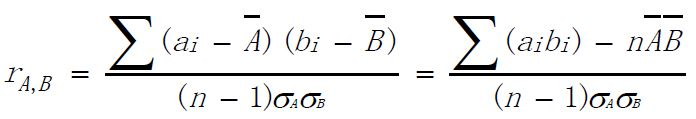### 相关关系度量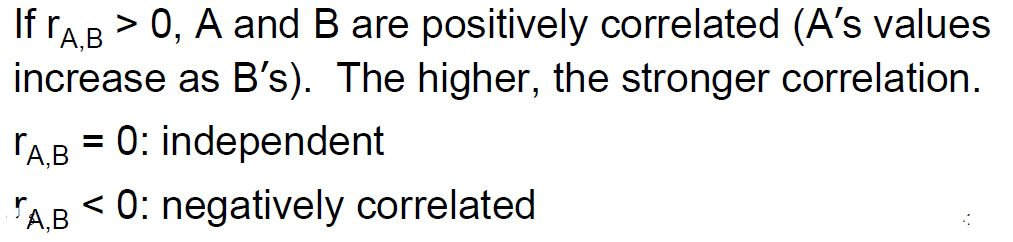### 皮尔逊相关系数的适用范围

1. 两个变量之间是线性关系，都是连续数据。
2. 两个变量的总体是正态分布，或接近正态的单峰分布。
3. 两个变量的观测值是成对的，每对观测值之间相互独立。

### 如何理解皮尔逊相关系数

rubyist：皮尔逊相关系数理解有两个角度(4)皮尔逊相关的约束条件

• 1 两个变量间有线性关系
• 2 变量是连续变量
• 3 变量均符合正态分布,且二元分布也符合正态分布
• 4 两变量独立

Note: lz文本很短，关键词很少的时候，余弦距离就很难计算出准确的相似度。这时候可以使用主题模型。

## 汉明距离-分类数据点间的距离Jaccard similarity 还可以用集合的公式来表达，这里就不多说了。

## kl散度/相对熵/kl距离

1. 相对熵（relative entropy）又称为KL散度（Kullback–Leibler divergence，简称KLD），信息散度（information divergence），信息增益（information gain）。

KL散度是两个概率分布P和Q差别的非对称性的度量（designed to measure the difference between probability distributions）。 KL散度是用来 度量使用基于Q的编码来编码来自P的样本平均所需的额外的位元数。 典型情况下，P表示数据的真实分布，Q表示数据的理论分布，模型分布，或P的近似分布。

### 定义$D_{\mathrm{KL}}(P\|Q) = \sum_i P(i) \ln \frac{P(i)}{Q(i)}. \!$

### 特性

1. 相对熵的值为非负数$D_{\mathrm{KL}}(P\|Q) \geq 0, \,$

2. 尽管从直觉上KL散度是个度量或距离函数, 但是它实际上并不是一个真正的度量或距离。因为KL散度不具有对称性：从分布P到Q的距离（或度量）通常并不等于从Q到P的距离（或度量）。$D_{\mathrm{KL}}(P\|Q) \neq D_{\mathrm{KL}}(Q\|P)$

L散度是不对称的，当然，如果希望把它变对称，

Ds(p1, p2) = [D(p1, p2) + D(p2, p1)] / 2

[KL散度]

[相对熵]

2. 概率分布之间的距离

### kl散度的python+scipy实现

{计算topic_word分布矩阵所有topic_word分布两两之间的相似度-kl散度}

1.

# 方法1（pdist只能计算对称kl散度）
topic_similar_mat = spatial.distance.pdist(tw_dist_ndaray,
metric=lambda P, Q: ((sum(kl_div(P, Q)) + sum(kl_div(Q, P))) / 2))

2.

def kl(P, Q):
'''
计算两个ndarray的kl散度
KL散度仅当概率P和Q各自总和均为1，且对于任何i皆满足Q(i)>0及P(i)>0时，才有定义
'''
return sum(P * log(P / Q)) if (P > 0).all() and (Q > 0).all() else None
# return sum(where((P > 0).all() and (Q > 0).all(), P * log(P / Q), None), axis=0)

#方法2（对称kl散度，自定义kl函数）
topic_similar_mat = spatial.distance.pdist(tw_dist_ndaray,
metric=lambda P, Q: ((kl(P, Q) + kl(Q, P)) / 2))

Note: kl散度可以通过scipy包的stats.entropy计算[Scipy教程 - 统计函数库scipy.stats ]

3.

# 方法3（非对称kl散度）
topic_similar_mat = zeros([len(tw_dist_ndaray), len(tw_dist_ndaray)])
for i_id, i in enumerate(tw_dist_ndaray):
for j_id, j in enumerate(tw_dist_ndaray):
if i_id != j_id:
topic_similar_mat[i_id, j_id] = stats.entropy(tw_dist_ndaray[i_id], tw_dist_ndaray[j_id])

4.

            topic_similar_mat[i_id, j_id] = sum(kl_div(tw_dist_ndaray[i_id], tw_dist_ndaray[j_id]))

Note：

1. 计算出的结果自己和自己的kl散度为0，为了排序计算与别人的相似度应该将对角线中的元素改为max

topic_similar_mat = spatial.distance.squareform(topic_similar_mat)
max_dist = topic_similar_mat.max()
for i in range(len(topic_similar_mat)):
topic_similar_mat[i, i] = max_dist+1
print(topic_similar_mat)

2. 非对称kl散度不是对称的，而用pdist计算出的topic_similar_mat一定是对称的，因为pdist只计算上三角，所以使用pdist必须要用对称的kl散度

## 闵可夫斯基距离$D_{\mathrm{KL}}(P\|Q) \neq D_{\mathrm{KL}}(Q\|P)$$D_{\mathrm{KL}}(P\|Q) \neq D_{\mathrm{KL}}(Q\|P)$ : 该维度上的均值$D_{\mathrm{KL}}(P\|Q) \neq D_{\mathrm{KL}}(Q\|P)$ : 该维度上的标准差

## 马氏距离Note: 这个也正是多维高斯分布的指数项的二次型，即高斯对于x的依赖的二次型表达。当$D_{\mathrm{KL}}(P\|Q) \neq D_{\mathrm{KL}}(Q\|P)$为单位矩阵时，马氏距离就变成了欧氏距离。import numpy as np
import pylab as pl
import scipy.spatial.distance as dist
def plotSamples(x, y, z=None):
stars = np.matrix([[3., -2., 0.], [3., 2., 0.]])
if z is not None:
x, y = z * np.matrix([x, y])
stars = z * stars
pl.scatter(x, y, s=10)    # 画 gaussian 随机点
pl.scatter(np.array(stars), np.array(stars), s=200, marker='*', color='r')  # 画三个指定点
pl.axhline(linewidth=2, color='g') # 画 x 轴
pl.axvline(linewidth=2, color='g')  # 画 y 轴
pl.axis('equal')
pl.axis([-5, 5, -5, 5])
pl.show()
# 产生高斯分布的随机点
mean = [0, 0]      # 平均值
cov = [[2, 1], [1, 2]]   # 协方差
x, y = np.random.multivariate_normal(mean, cov, 1000).T
plotSamples(x, y)
covMat = np.matrix(np.cov(x, y))    # 求 x 与 y 的协方差矩阵
Z = np.linalg.cholesky(covMat).I  # 仿射矩阵
plotSamples(x, y, Z)
# 求马氏距离
print '\n到原点的马氏距离分别是：'
print dist.mahalanobis([0,0], [3,3], covMat.I), dist.mahalanobis([0,0], [-2,2], covMat.I)
# 求变换后的欧几里得距离
dots = (Z * np.matrix([[3, -2, 0], [3, 2, 0]])).T
print '\n变换后到原点的欧几里得距离分别是：'
print dist.minkowski([0, 0], np.array(dots), 2), dist.minkowski([0, 0], np.array(dots), 2)

# 其它距离度量方法

mutual information

Spearman's rank coefficient

Earth Mover's Distance

## SimRank 相似：SimRank 迭代算法

SimRank来自图论，说两个变量相似，因为他们链接了同一个或相似的节点。

## 非欧氏距离及其满足公理性质的证明

### Jaccard Dist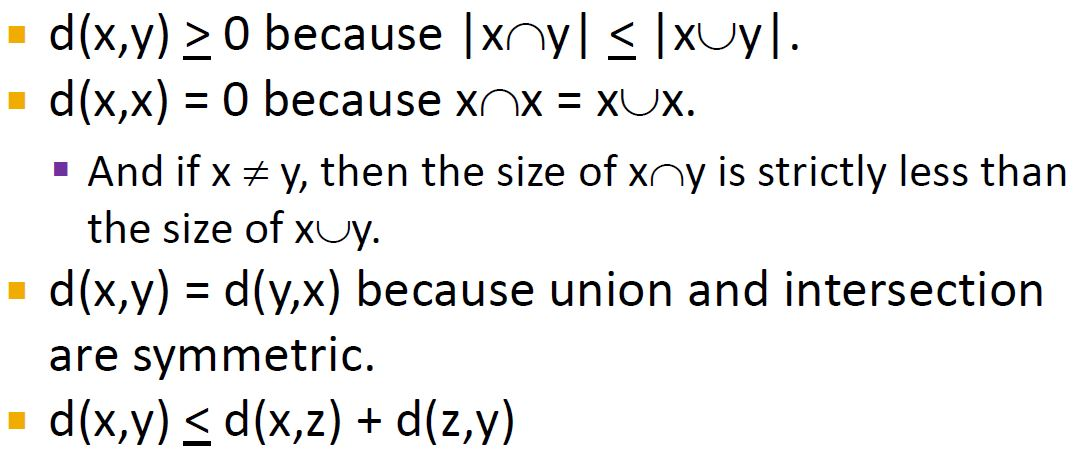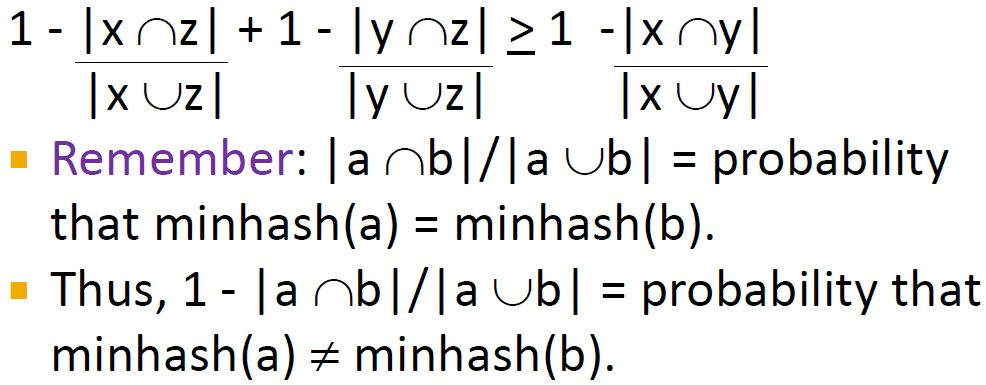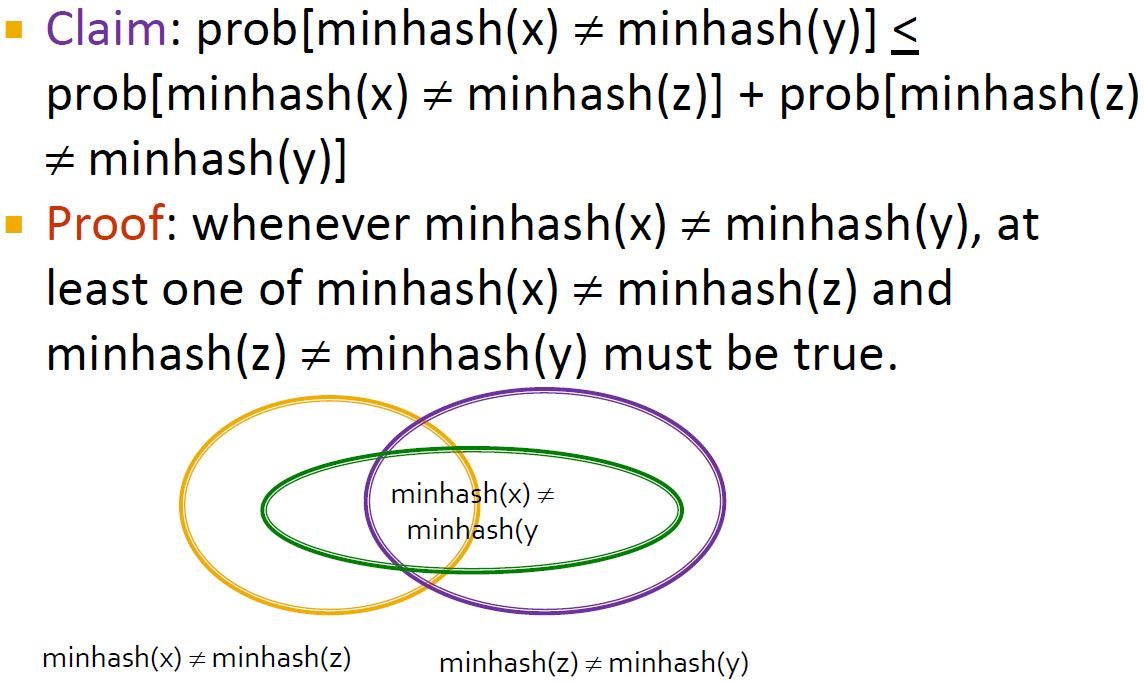Note: Proof中使用反证法：两个都不成立，即都相等时，minhash(x)=minhash(y)了。

### Cosine Dist余弦距离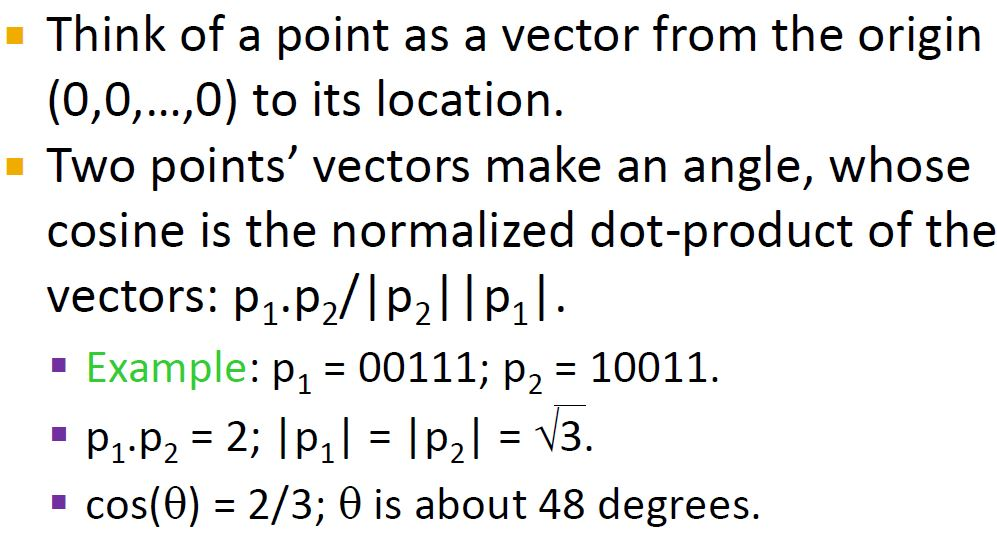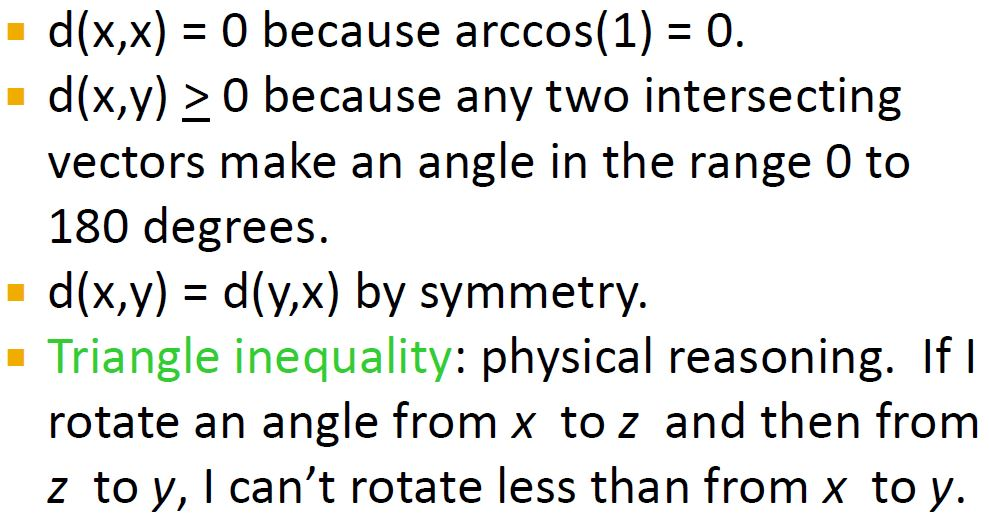Note: vectors here are really directions, not magnitudes.So two vectors with the same direction and different magnitudes are really the same vector.Even to vector and its negation, the reverse of the vector,ought to be thought of as the same vector.

### Edit distance编辑距离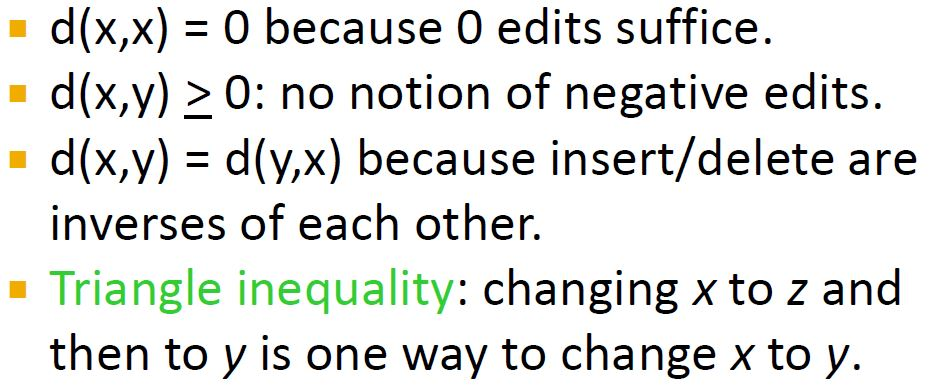### Hamming distance汉明距离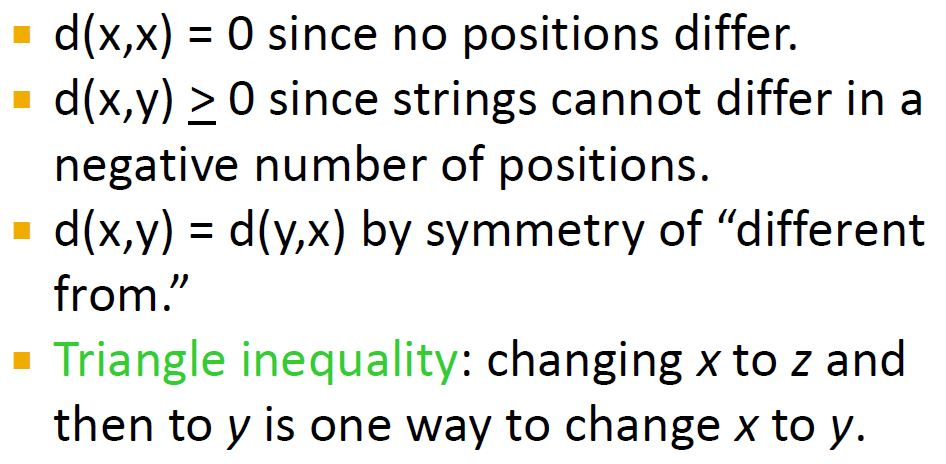ref: 如何计算两个文档的相似度

Machine Learning: Measuring Similarity and Distance

What is Mahalanobis distance?

Cosine similarity, Pearson correlation, and OLS coefficients

06-304万+11-14618
04-122339
10-302万+
01-052879
03-253478
12-295712
11-171万+
05-07305